# 1.5: Distillation

$$\newcommand{\vecs}{\overset { \rightharpoonup} {\mathbf{#1}} }$$ $$\newcommand{\vecd}{\overset{-\!-\!\rightharpoonup}{\vphantom{a}\smash {#1}}}$$$$\newcommand{\id}{\mathrm{id}}$$ $$\newcommand{\Span}{\mathrm{span}}$$ $$\newcommand{\kernel}{\mathrm{null}\,}$$ $$\newcommand{\range}{\mathrm{range}\,}$$ $$\newcommand{\RealPart}{\mathrm{Re}}$$ $$\newcommand{\ImaginaryPart}{\mathrm{Im}}$$ $$\newcommand{\Argument}{\mathrm{Arg}}$$ $$\newcommand{\norm}{\| #1 \|}$$ $$\newcommand{\inner}{\langle #1, #2 \rangle}$$ $$\newcommand{\Span}{\mathrm{span}}$$ $$\newcommand{\id}{\mathrm{id}}$$ $$\newcommand{\Span}{\mathrm{span}}$$ $$\newcommand{\kernel}{\mathrm{null}\,}$$ $$\newcommand{\range}{\mathrm{range}\,}$$ $$\newcommand{\RealPart}{\mathrm{Re}}$$ $$\newcommand{\ImaginaryPart}{\mathrm{Im}}$$ $$\newcommand{\Argument}{\mathrm{Arg}}$$ $$\newcommand{\norm}{\| #1 \|}$$ $$\newcommand{\inner}{\langle #1, #2 \rangle}$$ $$\newcommand{\Span}{\mathrm{span}}$$

## Introduction to Distillation

$$B$$ = mass or molar flow rate of the bottoms stream leaving the systems (mass time-1 or mole time-1)

$$D$$ = mass or molar flow rate of the distillate stream leaving the system (mass time-1 or mole time-1)

$$F$$ = mass or molar flow rate of the feed stream entering the system (mass time-1 or mole time-1)

$$L$$ = mass or molar flow rate of the liquid reflux returned to the column from the condenser (mass time-1 or mole time-1); also generic flow rate of the liquid phase in the rectifying section

$$\overline L$$ = mass or molar flow rate of the liquid leaving the bottom of the column and entering the reboiler (mass time-1 or mole time-1); also generic flow rate of the liquid phase in the stripping section

$$n$$ = generic stage number, stage 1 is at the top of the column

$$R$$ = reflux ratio

$$V$$ = mass or molar flow rate of vapor leaving the top of the column and entering the condenser (mass time-1 or mole time-1); also generic flow rate of the vapor phase in the rectifying section

$$\overline V$$ = mass or molar flow rate of the gaseous boilup returned to the column from the reboiler (mass time-1 or mole time-1); also generic flow rate of the vapor phase in the stripping section

$$x$$ = mass or mole fraction of the light key in a liquid stream

$$x_B$$ = mass or mole fraction of the light key in the bottoms stream

$$x_D$$ = mass or mole fraction of the light key in the distillate stream

$$x_n$$ = mass or mole fraction of the light key in the liquid leaving stage $$n$$

$$y$$ = mass or mole fraction of the light key in vapor stream

$$y_n$$ = mass or mole fraction of the light key in the vapor leaving stage $$n$$

$$z_F$$ = mass or mole fraction of the light key in the feed stream

Overall material balance

$F = D + B \tag{20.1}$

Material balance on light key

$F z_F = x_DD + x_BB \tag{20.2}$

Combination of material balances in Equations 20.1 and 20.2

$D = F \left( \dfrac{z_F - x_B}{x_D - x_B} \right) \tag{20.3}$

$R=\frac{L}{D} \tag{20.4}$

$V_B=\frac{\overline V}{B} \tag{20.5}$

Material balance on stages $$1-n$$, the rectifying section of the column

$y_{n+1}=\left(\frac{L}{V}\right)x_n+y_1-\left(\frac{L}{V}\right)x_0 \tag{20.6}$

Rectifying section operating line

$y_{n+1}=\left(\frac{R}{R+1}\right)x_n+\left(\frac{x_D}{R+1}\right) \tag{20.7}$

Stripping section operating line

$y_{n+1}=\left(\frac{V_B+1}{V_B}\right)x_n-\left(\frac{x_B}{V_B}\right) \tag{20.8}$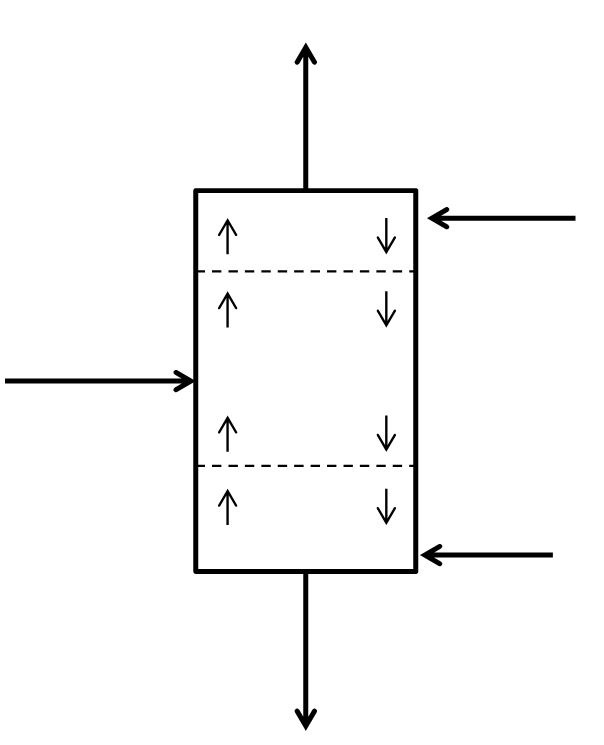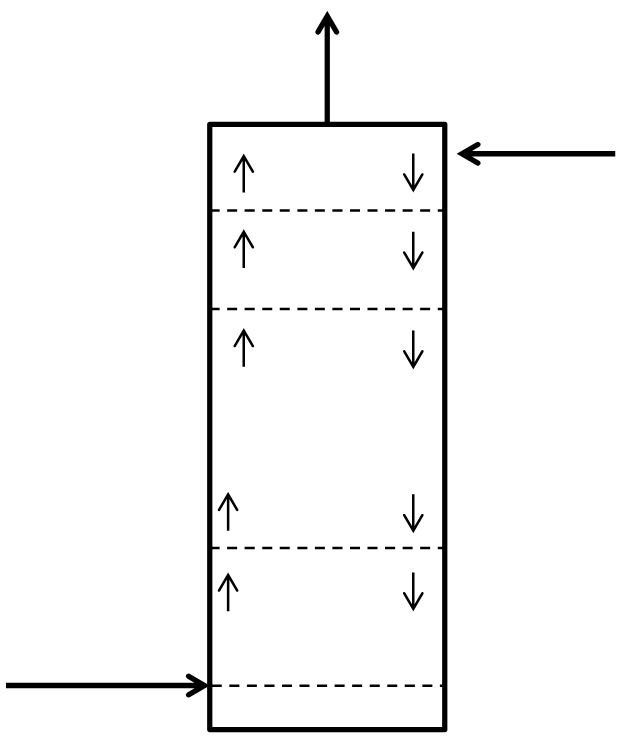## McCabe-Thiele Method for Finding N and Feed Stage Location

$$\Delta H^{\rm vap}$$ = enthalpy change of vaporization of the feed stream at the column operating pressure (energy mole-1)

$$C_{P_L}$$ = heat capacity of the liquid feed stream (energy mole-1 temperature-1)

$$C_{P_V}$$ = heat capacity of the vapor feed stream (energy mole-1 temperature-1)

$$F$$ = molar flow rate of the feed stream entering the system (mole time-1)

$$L$$ = molar flow rate of the liquid phase in the rectifying section (mole time-1)

$$\overline L$$ = molar flow rate of the liquid phase in the stripping section (mole time-1)

$$L_F$$ = molar flow rate of the liquid portion of the feed stream (mole time-1)

$$n$$ = generic stage number, stage 1 is at the top of the column

$$q$$ = metric that reflects the physical state of the feed stream (unitless)

$$R$$ = reflux ratio

$$T_b$$ = bubble-point temperature of the feed stream at the column operating pressure (temperature)

$$T_d$$ = dew-point temperature of the feed stream at the column operating pressure (temperature)

$$T_F$$ = temperature of the feed stream (temperature)

$$V$$ = molar flow rate of the vapor phase in the rectifying section (mole time-1)

$$\overline V$$ = molar flow rate of the vapor phase in the stripping section (mole time-1)

$$V_F$$ = molar flow rate of the vapor portion of the feed stream (mole time-1)

$$x_B$$ = mole fraction of the light key in the bottoms stream

$$x_D$$ = mole fraction of the light key in the distillate stream

$$x_n$$ = mole fraction of the light key in the liquid leaving stage

$$z_F$$ = mole fraction of the light key in the feed stream

### Finding the Theoretical Number of Stages from known Reflux Ratio, Boilup Ratio, Distillate Composition and Bottoms Composition

Rectifying section operating line

$y_{n+1}=\left(\frac{R}{R+1}\right)x_n+\left(\frac{1}{R+1}\right)x_D \tag{21.1}$

Stripping section operating line

$y_{n+1}=\left(\frac{V_B+1}{V_B}\right)x_n-\left(\frac{1}{V_B}\right)x_B \tag{21.2}$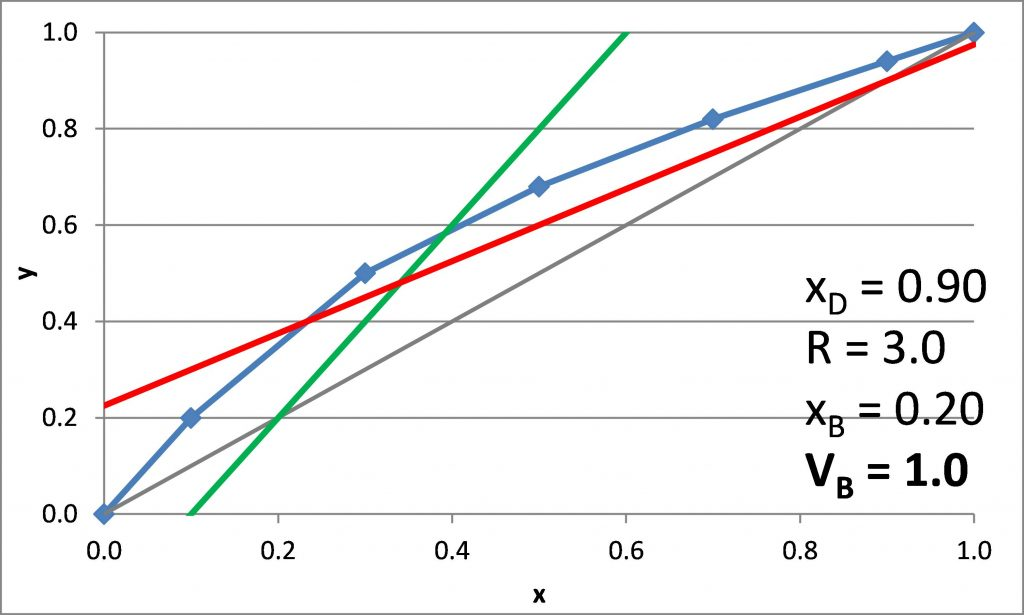### Plotting the q-line

$F = L_F + V_F \tag{21.3}$

$q=\frac{(\overline L -L)}{F}=1+(\frac{\overline V-V}{F}) \tag{21.4}$

For sub-cooled liquid, q > 1

$q=1+\frac{C_{P_L}(T_b-T_F)}{\Delta H^{\rm vap}} \tag{21.5}$

For a saturated liquid,

$q=1 \tag{21.6}$

For a mixture of liquid and vapor, 0 < q < 1

$q=\frac{L_F}{F} \tag{21.7}$

For a saturated vapor, q = 0

$q = 0 \tag{21.8}$

For sub-heated vapor, q < 0

$q=\frac{C_{P_V}(T_d-T_F)}{\Delta H^{\rm vap}} \tag{21.9}$

q-line

$y=\left(\frac{q}{q-1}\right)x-\left(\frac{1}{q-1}\right)z_F \tag{21.10}$

Watch this two-part video series from LearnChemE that demonstrates how to use the McCabe-Thiele graphical method to determine the number of equilibrium stages needed to meet a specified separation objective: McCabe-Thiele Graphical Method Example Part 1 (8:21): https://youtu.be/Cv4KjY2BJTA and McCabe-Thiele Graphical Method Example Part 2 (6:35): https://youtu.be/eIJk5uXmBRc

Watch this video from LearnChemE for a conceptual demonstration of how to relate stepping off stages to distillation column design: McCabe-Thiele Stepping Off Stages (7:02): https://youtu.be/rlg-ptQMAsg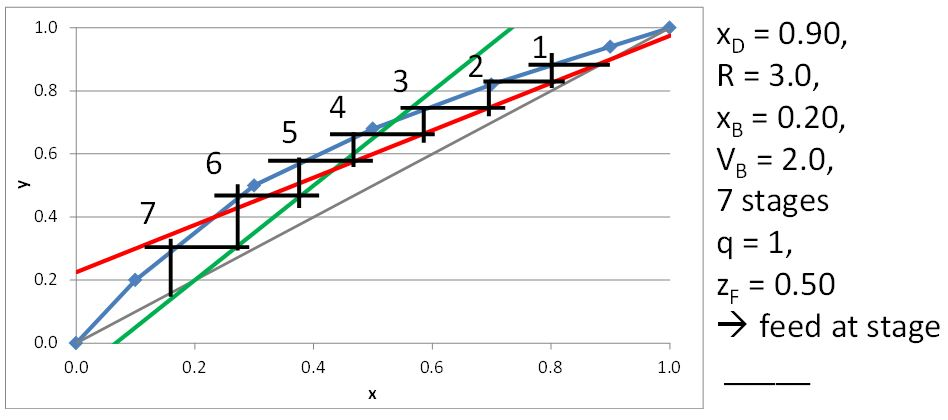## McCabe-Thiele Method for Finding the Minimum Number of Stages, the Minimum Reflux Ratio, and the Minimum Boilup Ratio

$$\alpha$$ = relative volatility of the light key and the heavy key at a given temperature (unitless)

$$\alpha_F$$ = relative volatility of the light key and the heavy key at the feed temperature (unitless)

$$\gamma_{HK}$$ = activity coefficient of the heavy key; can be a function of $$x$$ and/or $$T$$; 1 for an ideal solution (unitless)

$$\gamma_{LK}$$ = activity coefficient of the light key; can be a function of $$x$$ and/or $$T$$; 1 for an ideal solution (unitless)

$$B$$ = molar flow rate of the bottoms leaving the system (mol time-1)

$$D$$ = molar flow rate of the distillate leaving the system (mol time-1)

$$F$$ = molar flow rate of the feed stream (mol time-1)

$$L$$ = molar flow rate of liquid within the rectifying section, assumed constant in McCabe-Thiele model (mol time-1)

$$\overline L$$ = molar flow rate of liquid within the stripping section, assumed constant in McCabe-Thiele model (mol time-1)

$$N_{t,\rm min}$$ = minimum required number of theoretical stages for a given combination of equilibrium data, $$x_D$$ and $$x_B$$

$$P_{HK}^{\rm sat}$$ = saturated vapor pressure of the heavy key at a given temperature, i.e. by Antoine equation (pressure)

$$P_{LK}^{\rm sat}$$ = saturated vapor pressure of the light key at a given temperature, i.e. by Antoine equation (pressure)

$$q$$ = metric that indicates that physical state of the feed stream, i.e. $$q$$ = 1 for saturated liquid (unitless)

$$R$$ = reflux ratio = $$L/D$$ (unitless)

$$R_{\rm min}$$ = reflux ratio that requires an infinite number of stages in the rectifying section (unitless)

$$V$$ = molar flow rate of vapor within the rectifying section, assumed constant in McCabe-Thiele model (mol time-1)

$$\overline V$$ = molar flow rate of vapor within the stripping section, assumed constant in McCabe-Thiele model (mol time-1)

$$V_B$$ = boilup ratio = $$\overline V/B$$

$$V_{B,\rm min}$$ = boilup ratio that requires an infinite number of stages in the stripping section (unitless)

$$V_F$$ = molar flow rate of the vapor component of the feed stream (mol time-1)

$$x_B$$ = target mole fraction of the light key in the bottoms product

$$x_D$$ = target mole fraction of the light key in the distillate product

$$x_{HK}$$ = mole fraction of the heavy key in the liquid phase

$$x_{LK}$$ = mole fraction of the light key in the liquid phase

$$y_{HK}$$ = mole fraction of the heavy key in the vapor phase

$$y_{LK}$$ = mole fraction of the light key in the vapor phase

$$z_F$$ = mole fraction of the light key in the feed stream

$R_{\rm min}=\frac{(L/V)_{\rm min}}{1-(L/V)_{\rm min}} \tag{22.1}$

$$(L/V)_{\rm min}$$ = slope of the line that connects ($$x_D$$, $$x_D$$) to the intersection of the q-line and the equilibrium curve

$\alpha=\frac{y_{LK}/y_{HK}}{x_{LK}/x_{HK}}=\frac{\gamma_{LK}P_{LK}^{\rm sat}}{\gamma_{HK}P_{HK}^{\rm sat}} \tag{22.2}$

$V_{B,\rm min}=\frac{1}{(\overline L /\overline V)_{\rm max}-1} \tag{22.3}$

$$(\overline L/\overline V)_{\rm min}$$ = slope of the line that connects ($$x_B$$, $$x_B$$) to the intersection of the q-line and the equilibrium curve

$V_{B}=\frac{L+D-V_F}{B}=\frac{D(R+1)-V_F}{B} \tag{22.4}$

when $$q \leq 0$$, $$V_F = F$$; when $$0 < q < 1$$, $$V_F = (1-q)F$$; when $$q \geq 1$$, $$V_F = 0$$

*we will use Eq 22.4 to calculate $$V_B$$ as a function of our selected $$R$$

#### Example

$$x_D = 0.80$$, $$q = 0$$, $$z_F = 0.25$$

$$(L/V)_{\rm min}$$ =

$$R_{\rm min}$$ =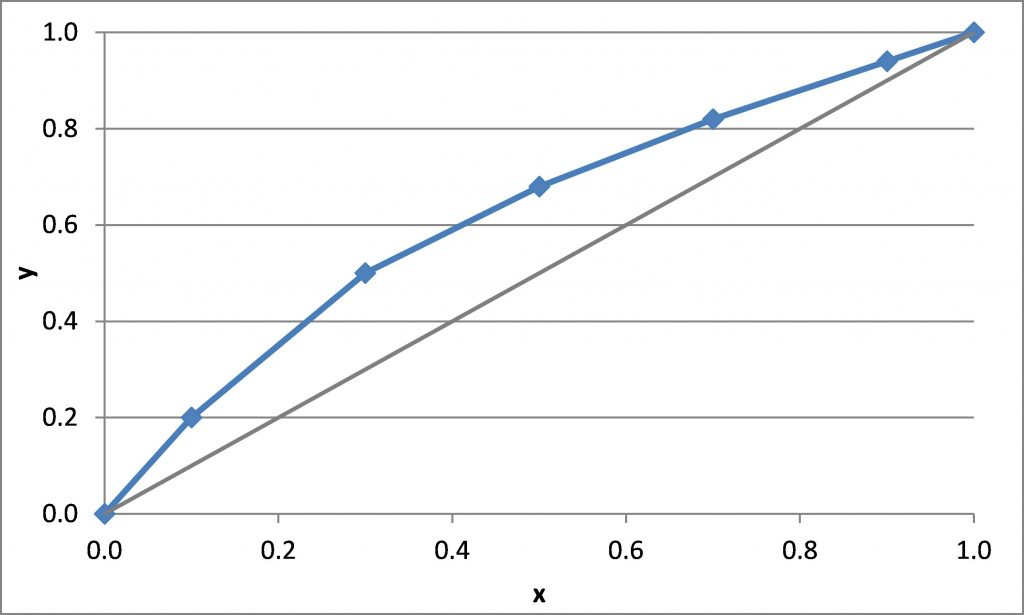#### Example

$$x_D = 0.90$$, $$x_B = 0.20$$

$$N_{t,\rm min} =$$## Distillation Energy Demand and Correlations for Efficiency

$$\alpha$$ = relative volatility of the light key and heavy key (unitless). For equation 23.2, this is evaluated at the average column temperature.

$$\Delta H^{\rm vap}$$ = average heat of vaporization for the stream entering the condenser or reboiler (energy mole-1)

$$\Delta H_S^{\rm vap}$$ = average heat of vaporization for the steam entering the reboiler (energy mole-1)

$$\mu$$ = liquid phase viscosity (cP). For eqs 23.1 and 23.2, this is the viscosity of the feed stream at the average column temperature.

$$B$$ = bottoms flow rate (mole time-1)

$$C_{P,\rm H_2O}$$ = heat capacity of liquid water (energy mole-1 temperature-1) or (energy mass-1 temperature-1)

$$D$$ = distillate flow rate (mole time-1)

$$E_O$$ = stage efficiency (unitless)

$$L$$ = liquid flow rate in the rectifying section (mole time-1)

$$\overline L$$ = liquid flow rate in the stripping section (mole time-1)

$$m_{\rm cw}$$ = flow rate of cooling water to condenser (mass time-1) or (mole time-1)

$$m_s$$ = flow rate of steam to reboiler (mass time-1) or (mole time-1)

$$Q_C$$ = energy demand (cooling) for the condenser (energy time-1)

$$Q_R$$ = energy demand (heating) for the reboiler (energy time-1)

$$R$$ = reflux ratio = $$L/D$$ (unitless)

$$T_{\rm in}$$ = temperature of cooling water entering the condenser (temperature)

$$T_{\rm out}$$ = temperature of cooling water leaving the condenser (temperature)

$$V_B$$ = boilup ratio = $$\overline V/B$$ (unitless)

$$V_F$$ = molar flow rate of the vapor portion of the feed (mole time-1)

### Correlations for Stage Efficiency

$E_O=13.3-68.8\log_{10}{\mu} \tag{23.1}$

Restrictions on eq 23.1: $$\mu = 0.066 – 0.355$$ cP, $$T = 157 – 420$$°F, $$P = 14.7 – 366$$ psia, $$E_O = 41 – 88$$%

O’Connell

$E_O=\frac{50.3}{(\alpha\mu)^{0.226}} \tag{23.2}$

when $$0.1 \leq \alpha \mu \leq 1$$, adjust $$E_O$$ calculated by 23.2 with correction factor from Table 7.5

Restriction on eq 23.2: $$\alpha = 1.16 – 20.5$$

### Condenser and Reboiler Energy Demand

total condenser

$Q_C=D(R+1)\Delta H^{\rm vap} \tag{23.3}$

partial reboiler

$Q_R=BV_B\Delta H^{\rm vap} \tag{23.4}$

for partially vaporized feed $$(0 < q < 1)$$ and total condenser

$Q_R=Q_C\left[1-\frac{V_F}{D(R+1)}\right] \tag{23.5}$

$m_{\rm cw}=\frac{Q_C}{C_{P,\rm H_2O}(T_{\rm out}-T_{\rm in})} \tag{23.6}$

if using saturated steam for the reboiler

$m_S=\frac{Q_R}{\Delta H_S^{\rm vap}} \tag{23.7}$

## Distillation Packed Column Depth

$$\rm HETP$$ = height of equivalent theoretical plates

$$H_{OG} = x$$ (length)

$$\lambda$$ = local slope of equilibrium curve/local slope of operating line

${\rm HETP}=H_{OG}\frac{\ln\lambda}{\lambda-1} \tag{24.1}$

#### Example

We aim to distill benzene and toluene to a distillate that contains 95 mol% benzene and a bottoms stream that contains 95% toluene. The feed stream is 100 kmol/hr of an equimolar mixture with q = 0.50. We will be operating at 1.0 atm, $$R/R_{\rm min}$$ of 1.8 with a packed column containing 25-mm metal Bialecki rings. Assume operating at 70% of the flooding velocity. What depth of packing is needed to achieve this separation?

• For Antoine equation of the form $$\log_{10}{p^*} = A – B/(T+C)$$, where $$T$$ is in °C and $$p^*$$ is in mmHg
• Benzene: $$A = 6.89$$, $$B = 1204$$, $$C = 220$$
• Toluene: $$A = 6.96$$, $$B = 1350$$, $$C = 220$$
• 25-mm metal Bialecki rings: $$a=210$$, $$\epsilon = 0.956$$, $$C_h =0.692$$, $$C_p =0.891$$, $$C_l =1.461$$, $$C_v =0.331$$, $$C_s =2.521$$
• Toluene: $${\rm MW} = 92.14$$, $$\rho_L = 0.87$$ g/mL, $$\mu_L = 0.590$$ cP, $$\sigma_L = 27.73$$ dyne/cm
• Benzene: $${\rm MW} = 78.11$$, $$\rho_L = 0.88$$ g/mL, $$\mu_L = 0.652$$ cP, $$\sigma_L = 28.88$$ dyne/cm
• $$D_L = 1.85*10^{-5}$$ cm2/s (Table 3.4, Seader)
• $$D_V = 0.0565$$ cm2/s (estimated via eq 3-36, Seader)
• $$\mu_V = 0.0133$$ cP, estimated from online gas viscosity calculator (LMNO Engineering) as a function of T (94°C)This page titled 1.5: Distillation is shared under a CC BY-NC 4.0 license and was authored, remixed, and/or curated by Monica Lamm and Laura Jarboe (Iowa State University Digital Press) via source content that was edited to the style and standards of the LibreTexts platform; a detailed edit history is available upon request.